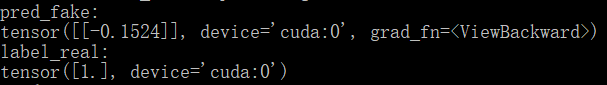# Dimension specified as 0 but tensor has no dimensions?

I load src_image and src_label from src_dataloader and convert them into variables. Then enter the classifier and use cross entropy to calculate the loss, but this error occurs. How should I solve it?

``````###### Classifier A ######

# Classifier loss
pred = netC_A(netG_A2B(real_A))
loss_C = criterion_class(pred, label_A)
loss_C.backward()

optimizer_C.step()
###################################
``````
``````for step, (src_image, src_label) in enumerate(src_dataloader):
real_A = make_variable(src_image)
label_A = make_variable(src_label.squeeze_())
``````
``````def make_variable(tensor):
"""Convert Tensor to Variable."""
if torch.cuda.is_available():
tensor = tensor.cuda()
return Variable(tensor)
``````

Either `pred` or `label_A` seem to be a scalar, while a tensor with at least one dimension is required.
Based on your code, I guess that `label_A` might be the scalar tensor, since you are `squeezing` it.

For a multi-class classification, the output should have the shape `[batch_size, nb_classes]` and the target `[batch_size]`.

Also, `Variables` are deprecated, so you should use tensors now.

Hi, ptrblck!
Both real_A and label_A are the images and labels that I loaded using ImageFolder. They should be in the form of tensors. So do I no longer need to use Variables to convert to variables?But I see that there are steps to convert variables in many similar codes. If I remove it, will pred or label_A still be able to enter the built network smoothly?
Can you tell me how to modify the code? Thank you!

They are most likely tensors, but the `squeeze_` operation could remove the single dimension as seen here:

``````x = torch.tensor()
print(x.shape)
> torch.Size()
print(x.squeeze().shape)
> torch.Size([])
``````

Could you explain, why `label_A = make_variable(src_label.squeeze_())` is needed?
Are you trying to squeeze a specific dimension? If so, you should use the `dim` argument.

These code snippets are also using the deprecated `Variables`.

Yes, you can simply pass tensors to the model.
If your input does not require gradients (which is the usual case), then just remove the `Variable(tensor)` call completely.

1. I originally wanted to use squeeze_ to convert the label into a one-dimensional tensor, but now I find that this is not necessary. So I removed squeeze_ and did not report the above error again.This is the label_A that I printed after removing squeeze_. Can it be used directly without the need to transform the form?But I received the following warning:

What caused this?
1. After I removed Variables, I encountered this error when entering the network. How do I deal with it?
``````preds = classifier(encoder(images))
``````

1. `nn.MSELoss` warns you, if your output and target shapes are different, as broadcasting will be applied, which might yield wrong results. If your output has the size `[batch_size, 1]`, make sure to pass the target is the same shape. Currently dim1 seems to be missing, so you might want to call `unsqueeze(1)` on the target.

2. The input tensor seems to be on the CPU, while a `CUDATensor` is expected, so you would need to push the tensor to the device.

PS: It’s better to post code snippets by wrapping them into three backticks ```, as it makes debugging easier.1. This is the code I used for `nn.MSELoss`. Does output and target shapes here refer to pred_fake and label_real in the code, respectively?I printed out their shapes.
``````fake_B = netG_A2B(real_A)
pred_fake = netD_B(fake_B)
loss_GAN_A2B = criterion_GAN(pred_fake, label_real)
``````
``````criterion_GAN = torch.nn.MSELoss()
``````2. In other words, if CUDA is available, then I need to push the input tensor from the CPU to the GPU. If CUDA is not used, the input tensor on the CPU can directly enter the network without the need to do Variable (tensor) processing, because the effect is the same, right?

PS: In addition to the code snippets, should the result of the operation also be wrapped into three backticks ```? To replace those pictures?

1. Yes, exactly. Your target has the shape ``, while the output uses `[1, 1]`, which will raise this warning. Just to make sure to avoid future issues, use `label_real = label_real.unsqueeze(1)` before passing it to `criterion_GAN`.

2. You have to push the model as well as all inputs to the right device. If you are using the GPU, you would thus have to transfer the data via `x = x.to('cuda')` or `x = x.cuda()`. To transfer it back, use `x = x.to('cpu')`. `Variables` are not needed anymore at all, neither for CUDA operations nor for CPU ops.

Yes, please post all code snippets and the outputs as formatted code, as it’s easier to debug and the forum search can index it. Pictures are not always easy to read, cannot be used to copy the source code for debugging, and the search won’t index it.

Thank you very much for your patience and detailed help! I understand most of what you said and solved the problem 1.
For question 2, I want to confirm whether the inputs form CPU is tensor or Variable(tensor) before using cuda? Or the two forms are the same, because I understand at what version it seems that Variable and tensor are merged?

Yes, they are the same since PyTorch `0.4`.
You can check the tensor directly, if it requires gradients etc, but there is no `Variable` usage anymore.

Ok, I see. Thanks again!